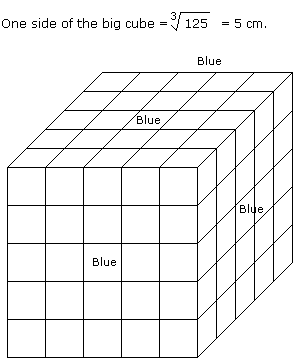# Verbal Reasoning - Cube and Cuboid

### Exercise :: Cube and Cuboid - Cube and Cuboid 6

All the faces of a cube are painted with blue colour. Then it is cut into 125 small equal cubes.

1.

How many small cubes will be formed having only one face coloured ?

 A. 54 B. 8 C. 16 D. 24

Explanation:No. of small cubes having only one face coloured = (5 - 2)2 x 6

= 9 x 6

= 54

2.

How many small cubes will be formed having no face coloured ?

 A. 27 B. 8 C. 16 D. 24

Explanation:No. of small cubes having no face coloured = (x - 2)3

= (5 - 2)3

= 27